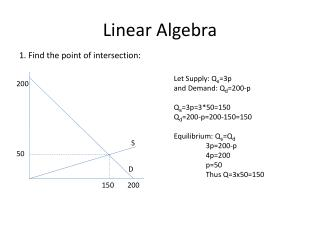DownloadDownload PresentationLinear Algebra

# Linear Algebra

Télécharger la présentation## Linear Algebra

- - - - - - - - - - - - - - - - - - - - - - - - - - - E N D - - - - - - - - - - - - - - - - - - - - - - - - - - -
##### Presentation Transcript

1. Linear Algebra 1. Find the point of intersection: Let Supply: Qs=3p and Demand: Qd=200-p Qs=3p=3*50=150 Qd=200-p=200-150=150 Equilibrium: Qs=Qd 3p=200-p 4p=200 p=50 Thus Q=3x50=150 200 S 50 D 150 200

2. Linear Algebra 2. Find line across two points: (x,y) = (5,10) and (x,y) = (25,20) Y=mX+b 10 = m5 + b 20 = m25 + b b = 10 - 5m 20 = 25m + (10-5m) 20 = 20m + 10 10 = 20m m = ½ b = 10 - 5m b = 10 - 5(1/2) b = 7.5 Therefore… P = ½Q + 7.5 or Q = 2P - 15 20 10 5 25